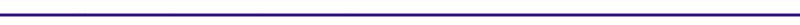Determination of Qin from the Fuel Heating ValueThe higher heating value(HHV) of the fuel will be assumed. This means that all the water products will be liquid. The HHV for each fuel is summarized in Table II, along with the air/fuel ratio for stoichiometric combustion.

Stoichiometric combustion occurs when all the oxygen atoms in the oxidizer react chemically to appear in the products. The oxidizer in this case is the air mixing with the fuel in the piston cylinder. The chemical reaction is given by: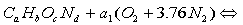Where:

a1,b1,c1,d1 = molar coefficients
a,b,c,d = fuel components

The stoichiometric fuel to air ratio can be calculated with:

FARs = 1 mole of fuel / a1(O2 + 3.76N2)

Table II. Fuel Properties

 Fuel Chemical Formula HHV [kJ/kg] [kg air/kg fuel] Air/Fuel Ratio Stoichiometric Fuel/Air Stoichiometric Methane CH4 55,500 17.2 0.05814 Propane C3H8 50,300 15.6 0.06410 Octane C8H18 47,900 15.1 0.06623 Methanol CH3OH 22,700 6.5 0.15385 Ethanol C2H5OH 29,700 8.99 0.11123 Hydrogen H2 141,600 27.2 0.03677 Gasoline C8H15 47,300 14.6 0.06849 Diesel C12.5H22.2 44,800 14.5 0.06897

In order to determine the heat input, Qin, in [J], the mass of the fuel entering the piston has to be calculated.

The equivalence ratio can be expressed as: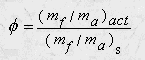If the equivalence ratio is given the above relation can be solved for the actual mass of the fuel.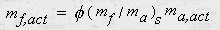The mass of the air, ma,actual, can be estimated from the displacement volume and the density.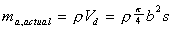Where:

b = bore
s = stroke
density of air = 1.2 kg/m3

The enrgy input, Qin, can be defined as:

Qin  =  HHVfuel * mf,actual

Two separate fuels can be tested to observe the changes in engine performance with the Engine Performance Applet with Fuel Type. This applet also includes the effect of heat transfer using Woschni's correlation.